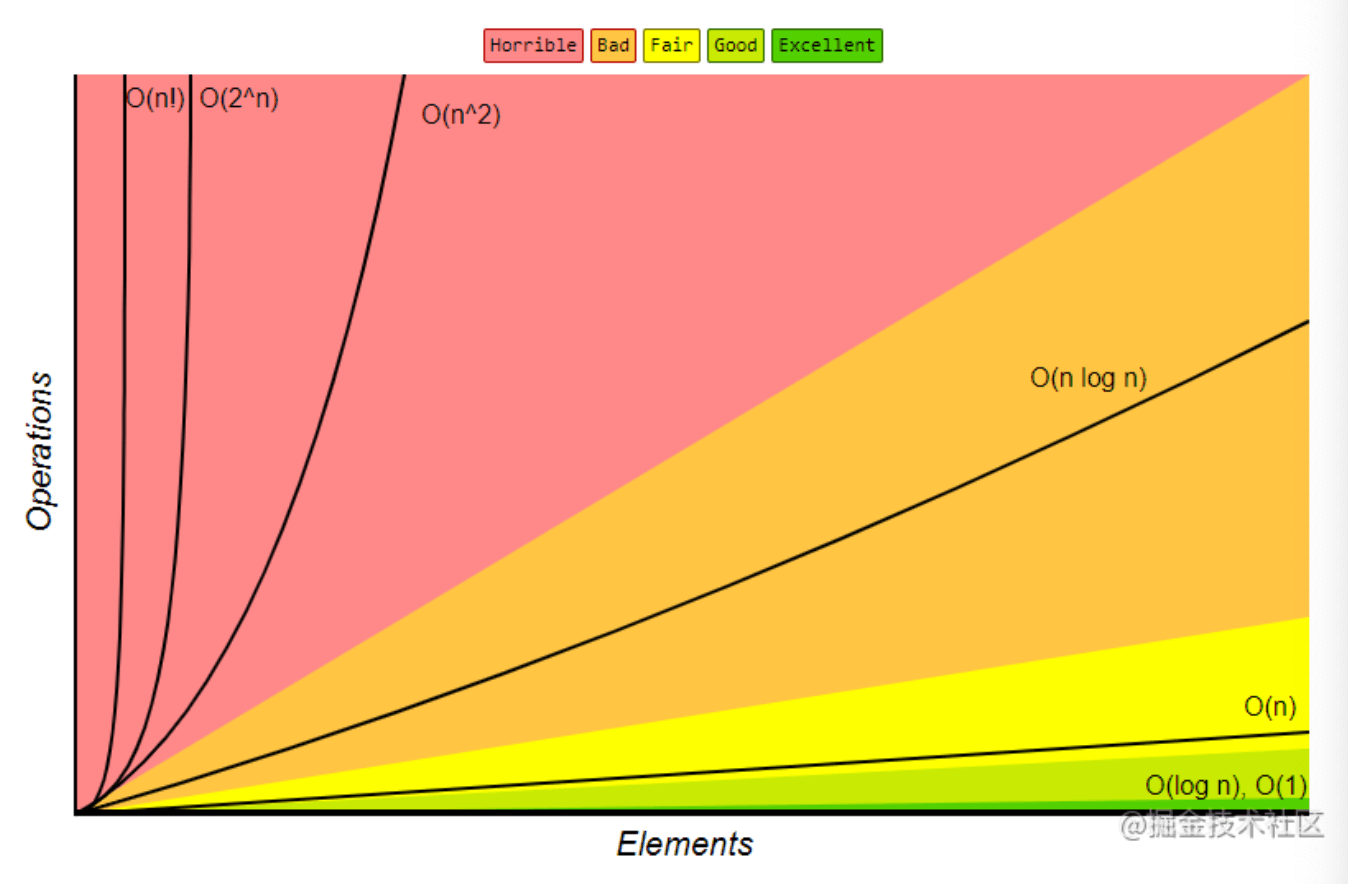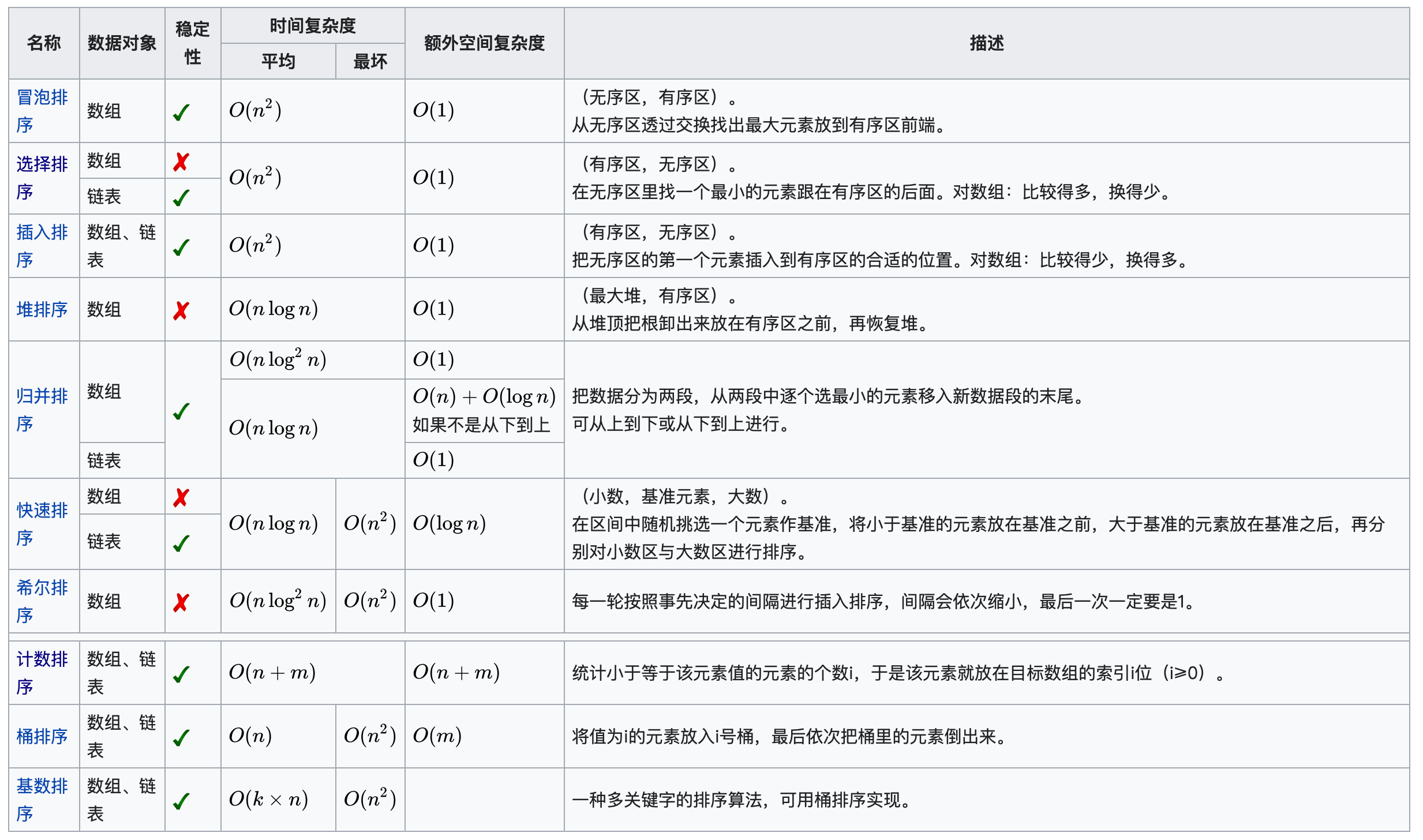# 【算法基础】算法复杂度

## 时间复杂度，空间复杂度

Posted by x-jeff on August 29, 2021

# 1.算法复杂度

## 1.1.时间复杂度

👉时间频度：一个算法执行所耗费的时间，从理论上是不能算出来的，必须上机运行测试才能知道（这种方式非常容易受运行环境的影响，在性能高的机器上跑出来的结果与在性能低的机器上跑的结果相差会很大。而且对测试时使用的数据规模也有很大关系）。但我们不可能也没有必要对每个算法都上机测试，只需知道哪个算法花费的时间多，哪个算法花费的时间少就可以了。并且一个算法花费的时间与算法中语句的执行次数成正比例，哪个算法中语句执行次数多，它花费时间就多。一个算法中的语句执行次数称为语句频度或时间频度，记为$T(n)$。算法的时间复杂度是指执行算法所需要的计算工作量。

1
2
3
4
5
6
7
8
9
10
11
12
13
# define n 100 // n 可根据需要定义,这里假定为100
void MatrixMultiply(int A[n][n], int B[n][n], int C[n][n])
{ //右边列为各语句的频度
int i, j, k; //1
for(i=0; i<n; i++) {  //n+1
for (j=0; j<n; j++) { //n*(n+1)
C[i][j] = 0; //n²
for (k=0; k<n; k++) {  //n²*(n+1)
C[i][j] = C[i][j] + A[i][k] * B[k][j];  //n³
}
}
}
}


$T(n)=2n^3+3n^2+2n+2$

1. 常数阶$O(1)$
2. 对数阶$O(log_2 n)$
3. 线性阶$O(n)$
4. 线性对数阶$O(nlog_2 n)$
5. 平方阶$O(n^2)$
6. 立方阶$O(n^3)$
7. $k$次方阶$O(n^k)$
8. 指数阶$O(2^n)$

## 1.2.空间复杂度

1. 存储算法本身所占用的存储空间：存储算法本身所占用的存储空间与算法书写的长短成正比，要压缩这方面的存储空间，就必须编写出较短的算法。
2. 算法的输入输出数据所占用的存储空间：算法的输入输出数据所占用的存储空间是由要解决的问题决定的，是通过参数表由调用函数传递而来的，它不随本算法的不同而改变。
3. 算法在运行过程中临时占用的存储空间：算法在运行过程中临时占用的存储空间随算法的不同而异，有的算法只需要占用少量的临时工作单元，而且不随问题规模的大小而改变，我们称这种算法是“就地”进行的，是节省存储的算法，有的算法需要占用的临时工作单元数与解决问题的规模$n$有关，它随着$n$的增大而增大，当$n$较大时，将占用较多的存储单元，例如快速排序和归并排序算法就属于这种情况。

✍️例子一：

1
2
3
int i = 1;
int j = 2;
int k = 1 + 2;


✍️例子二：

1
2
3
4
5
6
int j = 0;
int[] m = new int[n];
for (int i = 1; i <= n; ++i) {
j = i;
j++;
}


## 1.3.评估算法复杂度# 2.排序算法的算法复杂度• 均按从小到大排列。
• $k$代表数值中的“数位”个数。
• $n$代表数据规模。
• $m$代表数据的最大值减最小值。

# 3.参考资料